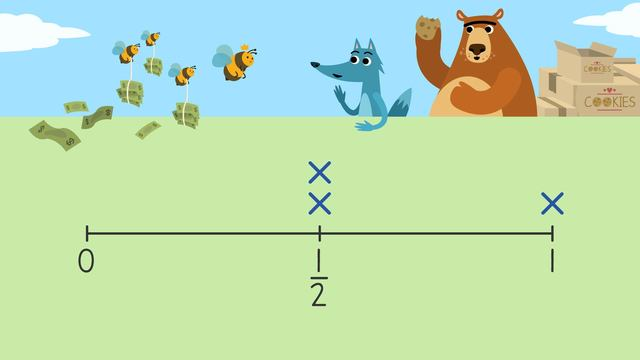# Creating Line Plots

Content Creating Line PlotsRating

Ø 3.9 / 23 ratings
The authorsTeam Digital
Creating Line Plots
CCSS.MATH.CONTENT.3.MD.B.4

## How to Make a Line Plot

What is a line plot in math? A line plot is a way of collecting data on a number line! Let's learn how to make a line plot by looking at how to create a line plot using collected data. The illustration below shows the necessary steps for creating a number line.## Creating Line Plots – Example

Let’s look at an example on how to create a line plot. Remember the necessary steps. First, draw a number line, as shown below.Then, identify the data range.

Box type Amount
$\frac{1}{4}$ 2
$\frac{1}{2}$ 2
$\frac{3}{4}$ 4
1 3

For this data, our range is one-quarter to one whole, so we will mark zero and one at either end of our number line. We use zero because almost all charts begin with zero, even when there is not a zero in the data.Next, include plots on the number line for the rest of the data range. We need to mark one-quarter, one-half, and three-quarters.Next, mark all the data on the line plot using an X to represent each box sold.Finally, create a title that tells the audience what your data is showing.## Creating Line Plots – Summary

Creating line plots is a useful way to represent your data. You can use it to show and analyze your data. To create line plots, follow the steps in the chart below:

Step # What to do
1 Draw a number line
2 Identify the data range
3 Identify and mark the
frequency of each data
point on the line plot
4 Mark the data on the line plot
5 Write a title

How do you draw a line plot step by step? What is a line plot example? How do you make a good line plot? This text gave you all the necessary information to represent collected data on a line plot. For extra practice, have a look at our interactive exercises, worksheets and further activities for 3rd grade after watching the video!

### TranscriptCreating Line Plots

1.this is cool I love it!!!!!!!!!!!!!!!!

From Noelani, about 1 month ago
2.man

From Carson (CJ), 2 months ago
3.yes

From Carson (CJ), 2 months ago
4.SO COOL

From Cameron, 7 months ago
5.FUNY

From Allison, 7 months ago